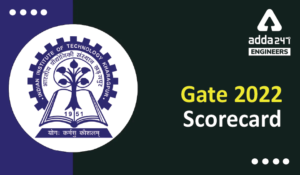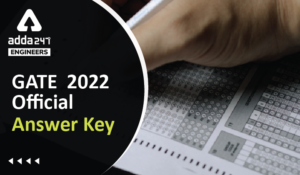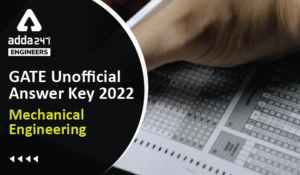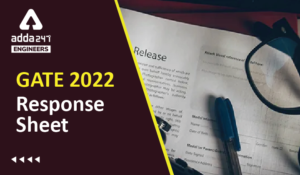Engineering Jobs   »   Electrical Engineering quizs   »   GATE 2022, ELECTRICAL GATE QUIZ,

# GATE’22 EE: Daily Practices Quiz 09-Oct-2021

## GATE 2022

GATE 2022 Important Dates: Organizing Institute for GATE 2022 is the Indian Institute of Technology Kharagpur. For GATE 2022 Exam IIT Kharagpur has released the official notification and Important dates regarding the GATE 2022 Exam. Aspirants preparing for GATE 2022 must check the details regarding GATE 2022 Important Dates. Read the complete article below for the information related to GATE 2022 Important dates.

## GATE’22 EE: Daily Practices Quiz

GATE’22 EE: Daily Practices Quiz 09-Oct-2021

Each question carries 2 marks
Negative marking: 0.66 mark

Total Questions: 10
Total marks: 20
Time: 20 min.

Q1. A co-axial cable of inner radius a and outer radius b. The space between conductor is filled with dielectric of permittivity ∈ and length of cable 4m. what is the capacitance of the cable?
(a) (2π∈)/ln⁡(b/a)
(b) ln⁡(b/a)/(2π∈)
(c) (π∈)/(2 ln⁡(b/a) )
(d) (8π∈)/ln⁡(b/a)

Q2. In a two-unit insulator string, voltage across the unit near to line conductor is 18.5 kV and string efficiency is 75%. The voltage of line conductor will be:
(a) 37 kV
(b) 48.07 kV
(c) 27.75 kV
(d) 30 kV

Q3. A step-down chopper connected to a d.c. supply, supplies a separately excited d.c. motor at a duty ratio of α. The ripple factor in the terminal voltage of the motor assuming continuous current conduction is-
(a) α/(1-α)
(b) (1-α)/α
(c) √((1-α)/α)
(d) √(α/(1-α))Q5. In a three-phase induction motor, the ratio power across air gap, rotor copper loss and gross mechanical power output is
(a) PG:sPG:(1-s) PG
(b) PG:sPG:(s-1) PG
(c) PG:(1-s) PG:sPG
(d) PG:sPG:(1+s) PGQ7. Consider a network function H(s)= (2(s+3))/((s+2)(s+4)). What is the steady state response due to step input?
(a) ½
(b) ¾
(c) 2
(d) 1

Q8. Which of the following is a non-maskable interrupt of 8085 microprocessors?
(a) INTR
(b) TRAP
(c) RST 6.5
(d) RST 7.5

Q9. For a JFET I_DSS = 8 mA and peak voltage V_p = –8V, what will be the drain current for gate to source voltage of –2V?
(a) 4.5 mA
(b) 8 mA
(c) 16 mA
(d) 12 mA

Q10. Determine the deflection factor (in V/m) of a CRO, when the deflection sensitivity of the CRO is 5 m/V.
(a) 0.25
(b) 0.35
(c) 0.20
(d) 0.40

## SOLUTIONSS5. Ans.(a)
Sol. In a three-phase induction motor, the ratio power across air gap, rotor copper loss and gross mechanical power output is-
P_G= Air gap power
sP_G = rotor copper loss
P_G (1-s) = gross mechanical power
So, ratio is-
P_G:sP_G ∶(1-s) P_G

S6. Ans.(c)
Sol. Peak current=I_0+(△i_c)/2=5+1.6/2=5.8 A

S7. Ans.(b)
Sol. steady state response=lim┬(s→0)⁡〖sC(s)=〗 lim┬(s→0)⁡= s×(2(s+3))/((s+2)(s+4))×1/s=3/4

S8. Ans.(b)
Sol. The hardware vectored interrupts are classified in to maskable and non-maskable interrupts.
RST 7.5, RST 6.5, RST 5.5 and INTR are maskable interrupt.

S9. Ans.(a)
Sol. I_D=I_DSS (1-V_GS/V_P )^2=8×10^(-3) (1-(-2)/(-8))^2=4.5 mA

S10. Ans.(c)
Sol. Deflection factor=1/(deflection sensitivity)=1/5=0.2 V/m.

Sharing is caring!

Thank You, Your details have been submitted we will get back to you.
•HURL Recruitment 2022 Apply Online for 1...
•GATE 2022 Scorecard Released, Check Step...
•GATE Result 2022 OUT Live Check Your GAT...
•GATE 2022 Official Answer Key Out, Downl...
•GATE 2022 Answer Key Mechanical Engineer...
•GATE 2022 Response Sheet, Direct Link to...